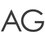# Hi Brilliantians!

Today I have developed a general formula for the classic Irodov problem-

3 particles are kept at the vertices of an equilateral triangle of side $a$.The velocity vector of the first particle continually points towards the second,the second towards the third and the third towards the first.After what time will they meet.Each moves with a constant speed $v$.

Well for a regular $n$ sided polygon ,

# $\boxed{T = \frac{a}{v_{0}(1-cos\frac{2\pi}{n})}}$

Working :

Well the usual stuff,figuring out relative velocities and then dividing the initial distance by the $v_{rel}$ to get the time.Becuase the trajectory forms logarithmic spirals and maintains its symmetricity all throughout the motion.

Note that each angle of the polygon is given by $\frac{(n-2)\pi}{n}$ therefore the exterior angle will be $\frac{2\pi}{n}$.Resolving the velocity component along this direction,we get

$v_{rel} =$ $v - v cos$ $\theta$ where $\theta$ $=$ $\frac{2\pi}{n}$

hence $t =$ $\frac{a}{v_{rel}}$ giving us our desired expression.

Note that for ,

$n = 3$ we have $t = 2a/3v$.

$n = 4$ we have $t = a/v$.

$n = 6$ we have $t = 2a/v$

$n = 12$ we have $t =$ $\frac{2a}{(2-\sqrt 3)v}$

$n = infinity$ we have $t =$ $infinity$ because now the path is a circle.

and so on.Note by Ayon Ghosh
3 years, 9 months ago

This discussion board is a place to discuss our Daily Challenges and the math and science related to those challenges. Explanations are more than just a solution — they should explain the steps and thinking strategies that you used to obtain the solution. Comments should further the discussion of math and science.

When posting on Brilliant:

• Use the emojis to react to an explanation, whether you're congratulating a job well done , or just really confused .
• Ask specific questions about the challenge or the steps in somebody's explanation. Well-posed questions can add a lot to the discussion, but posting "I don't understand!" doesn't help anyone.
• Try to contribute something new to the discussion, whether it is an extension, generalization or other idea related to the challenge.

MarkdownAppears as
*italics* or _italics_ italics
**bold** or __bold__ bold
- bulleted- list
• bulleted
• list
1. numbered2. list
1. numbered
2. list
Note: you must add a full line of space before and after lists for them to show up correctly
paragraph 1paragraph 2

paragraph 1

paragraph 2

[example link](https://brilliant.org)example link
> This is a quote
This is a quote
    # I indented these lines
# 4 spaces, and now they show
# up as a code block.

print "hello world"
# I indented these lines
# 4 spaces, and now they show
# up as a code block.

print "hello world"
MathAppears as
Remember to wrap math in $$ ... $$ or $ ... $ to ensure proper formatting.
2 \times 3 $2 \times 3$
2^{34} $2^{34}$
a_{i-1} $a_{i-1}$
\frac{2}{3} $\frac{2}{3}$
\sqrt{2} $\sqrt{2}$
\sum_{i=1}^3 $\sum_{i=1}^3$
\sin \theta $\sin \theta$
\boxed{123} $\boxed{123}$

Sort by:

This problem was already posted by Deepanshu Gupta in 2015 or 2014. :)

- 3 years, 5 months ago

Great problem and extension!

- 3 years, 5 months ago

Thanks a lot !

- 3 years, 5 months ago

Wow that was fast. Helped me on Aops Physics, was doing this problem :)

- 3 years, 5 months ago

Great generalization!

- 3 years, 1 month ago

Even though this is well known. XD

- 2 years, 8 months ago